Next: Implementation Up: Continuation 1D Previous: Continuation 1D   Contents

Mathematical background

Let consider a system ofequations in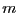unknowns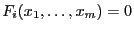and assume that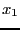may vary in the range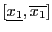and that it is considered as the parameter of this system. Using the solving algorithms of ALIAS (or any other method) we are able to determine the real roots of this system, if any, for a given value of the parameter, for example for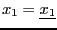. Let assume that we have found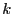real roots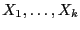. Assume now that we change the value ofto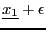. Using Kantorovitch theorem (see section 3.1.2) we are able to determine if Newton method with as initial estimate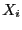will converge toward a solution of the new system and the radius of convergence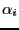such that the solution is unique in the ball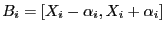(alternatively we may also use Moore theorem, see section 2.10). If this is the case and if the intersection of the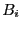is empty, then we are able to compute in a certified way the newsolutions of the new system. Otherwise we will repeat the procedure with a smaller value for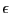until the method succeed. A failure case will be when the system become singular at a point (for example when 2 branches are collapsing). If this happen we will change the value ofuntil we find a new set of solutions which satisfy Kantorovitch theorem and start again the process.

This method enable to follow all the branches for which initial points have been found when solving the initial system. We may then store these points in an array, for example at regular step for the value of the parameter.

By default we use Kantorovitch theorem to follow the branches but by setting the global variable
ALIAS_Use_Kraw_Continuation to 1 we will use Moore test.Next: Implementation Up: Continuation 1D Previous: Continuation 1D   Contents
Jean-Pierre Merlet 2012-12-20